# Catch up on the latest AI articles# ReLabel, A Method For Relabeling ImageNet With Multiple Labeling Using Local Labels!

3main points
✔️Proposed ReLabel, a method for multi-class relabeling in ImageNet
✔️Also proposed LabelPooling, a method for discriminative learning of multi-class labels
✔️ ReLabel works well on tasks such as benchmarking ImageNet, transfer learning, and multiple label identification

written by Sangdoo YunSeong Joon OhByeongho HeoDongyoon HanJunsuk ChoeSanghyuk Chun
(Submitted on 13 Jan 2021)
Comments:
15 pages, 10 figures, tech report

## First of all

ImageNetImageNet is a very useful dataset for many tasks in computer vision and has a huge number of classes. However, as you will see when you try to use ImageNet in practice, ImageNet images contain multiple categories and many classes that are very confusing (e.g., dog types). Therefore, when training with ImageNet, other classes may be output or recognized as similar classes. For this reason, recent research has attempted to perform multiple labeling on ImageNet ( Lucas et al., Vaishaal et al. ), and evaluation methods for multiple labels have been proposed. Furthermore, we have found that our methods underperform human performance in the discrimination task on multi-label datasets ( Qizhe et al., Huga et al.).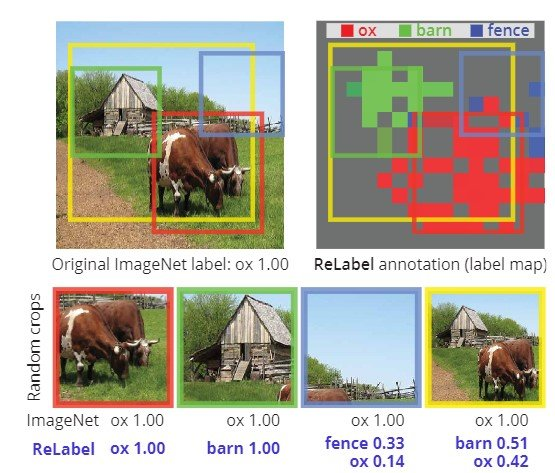Figure 1: Re-labeling of ImageNet training data.

The problem of assigning only a single label to data with multiple objects affects not only the evaluation but also the training, adding noise to the dataset. In particular, random cropping may be used for padding, which may include different objects in the cropped image, as shown in Figure 1, or random cropping may be used to crop areas where no object is present, even when only one object is present. Experiments show that using the ImageNet training set, 8% of the images are not cropped correctly when the random crop is used, and only 23.5% of the images have correct boxes and IoUs greater than 50% (Figure 2).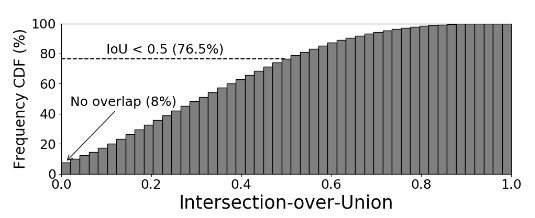Figure 2: Cumulative distribution on Intersection-over-Union (IoU)

Therefore, new labeling is performed for multiple labeling. Since manual labeling takes a huge amount of time for 1.28 million ImageNet samples, we propose ReLabel as a relabeling method in this work, which enables pixel-wise labeling for multiple labels and local labels. In the following, we describe labeling using ReLabel and LabelPooling, which outputs the correct answer data for multiple labels by pooling labeling scores for each random crop sample.

## Proposed method: ReLabel

The authors proposed a relabeling method, " ReLabel ", to output pixel-level correct labels for ImageNet training sets. There are two types of target label maps.

1. multi-class label
2. local label

The label map is based on the SoTA image discriminator trained on additional data, and in order to train the image discrimination model with local multiple labels, we propose a learning framework " LabelPooling " to create label maps. In the following, we will explain each of them.

### Re-labeling ImageNet

First of all, in order to get the correct label, because of the huge cost of manual labeling, we use a huge dataset such as JFT-300M and InstagramNet-1B, titled super-ImageNet, to train an image discriminator, and then we use ImageNet By fine-tuning, we create a learning model that can predict the class of ImageNet.

One thing worth mentioning here is that ImageNet is trained to output a single label (using softmax cross-entropy loss), but it also predicts multiple labels when there are multiple categories in the image. The reason is that cross-entropy loss is used as the loss function of the discriminator, and the correct category and image are given as input, and in addition, other labels are present. Then, to distinguish it from other labels, the cross-entropy loss is given as follows.

\begin{equation}-\frac{1}{2}(\Sigma_k y^0_k \log p_k(x) + \Sigma_k y^1_k \log p_k(x)) = - \Sigma_k \frac{y^0_k + y^1_k}{2} \log p_k(x)\end{equation}

where $y^c$ is the one-hot vector with 1 at index $c$, and $p(x)$ represents the prediction vector for image $x$.

In order to minimize this cross-entropy loss, it is when $p=q$ for $-\Sigma_k q_k \log p_k$, which is when the prediction is made using $p(x) = (\frac{1}{2}, \frac{1}{2})$. Where $q$ is the label (1 or 0) and $p$ is the prediction vector to be labeled. This allows a model with a single-label cross-entropy loss to output multiple labels if there is multiple label noise in the dataset.

In addition, we can use this discriminator to obtain local labels. By eliminating the global average pooling layer in the regular discriminator and adding a linear layer to the 1 x 1 convolutional layer, the discriminator acts as a fully coupled network (Bolei et al., Jonathan et al. ) The output of the model is $f(x) \in \mathbf{R }^{WxHxC}$ and the output $f(x)$ is used as a label map annotation $L \in \mathbf{R}^{WxHxC}$. The detailed figure is as follows.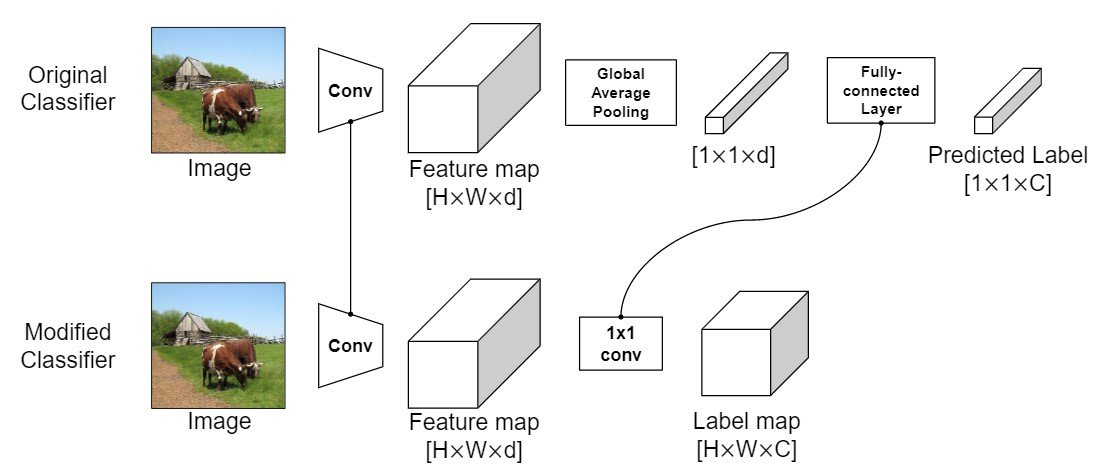Figure 3: Creating a label map

The label map output by this discriminator is shown below. The figure shows the heatmap for the top-2 category.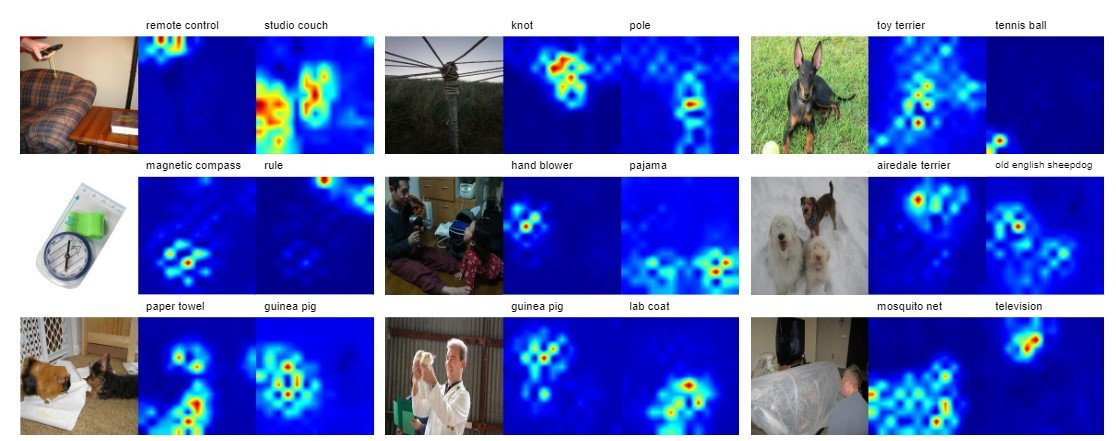Figure 4: Example of a label map

### Learning an Image Discriminator with Multiple Labels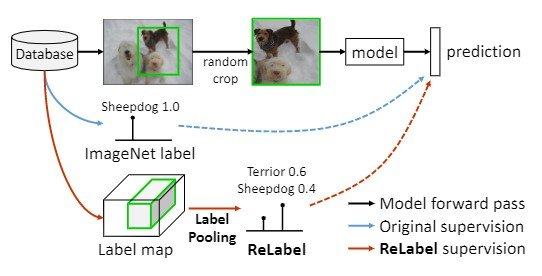Figure 5: Illustration of LabelPooling

As a method for learning with multiple labels $L \in \mathbf{R}^{WxHxC}$, this study proposes LabelPooling; LabelPooling supports multiple data by considering local correct data (Fig. 3).

In a typical ImageNet training setup, a single label per image is used to randomly crop the image. LabelPooling, on the other hand, uses a pre-defined label map to perform regional pooling on the regions corresponding to the random cropping. (ROIAlign regional pooling approach) Global average pooling and softmax are used to predict multiple labels. The pseudo-code is as follows.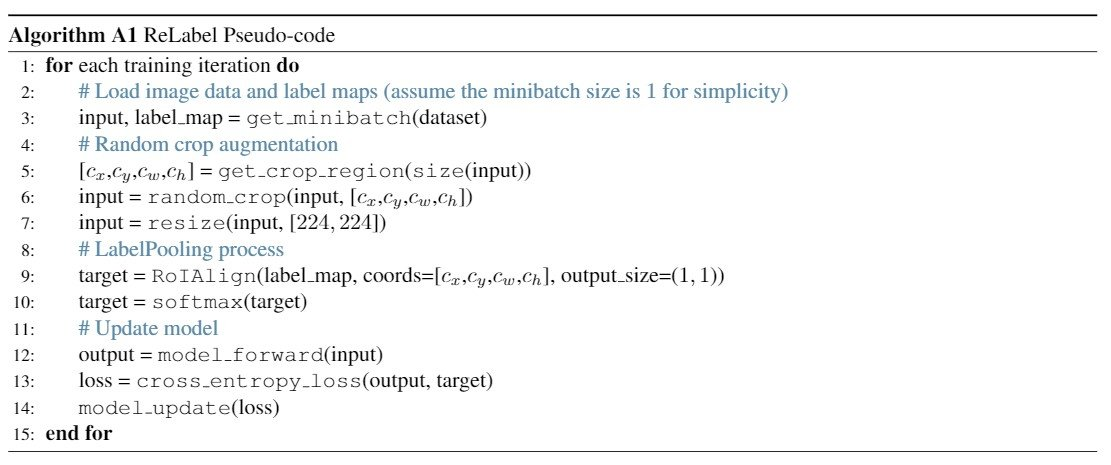Figure 6: Pseudo code of ReLabel

## Experiments and Results

We experiment with the proposed local multiple label labeling and learning with it on various tasks. To compare multiple network structures and evaluation metrics, we perform image recognition using ReLabel on ImageNet. We then evaluate the performance by performing transfer learning on models trained with ReLabel on the image detection, instance segmentation, and image identification tasks, and further prove that ReLabel produced good accuracy on the multiple label identification task on the COCO dataset. All experiments are performed using PyTorch on the NAVER Amart Machine Learning (NSML) platform below.

### Image Identification in ImageNet

ImageNet-1K Benchmark is used to evaluate ReLabel. The dataset contains 1.28 million training sets, 50,000 evaluation images, and 1000 categories. For data padding, we use random cropping, flipping, and color jittering. We start with a learning rate of 0.1 and train for 300 epochs using SGD. The batch size is 1024 and the weight decay rate is 0.0001.

#### Comparison of labeling methods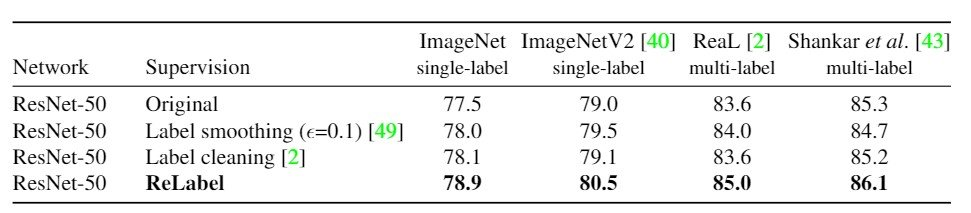Table 1: Identification of ImageNet

As a first experiment, we compare Label smoothing with other labeling methods: Labelsmoothing uses a small weight $(1-\epsilon)$ for the front class and $\epsilon$ for the back class. Label cleaning is a method that removes data from the training sample if the prediction of the teacher discriminator is different from the annotation of the correct answer data. In this study, we use the refined dataset after label cleaning and experiment with ReLabel on ResNet-50. The results are shown in Table 3, where we determine the accuracy of a single label on the evaluation datasets of ImageNet and ImageNetV2. Also, to find the accuracy of multiple labels, here we use the ReaL and Shankar datasets for comparison.

The evaluation method is $\frac{1}{N}\Sigma^N_{n=1} 1 (\argmax f(x_n) \in y_n)$ where $1(\cdot )$ is the indicator function and $\argmax f(x_n)$ is the top-1 prediction of model $f$. $N$ is the number of classes. The correct data for image $x_n$ is represented by $y_n$. The table shows that ReLabel is the most accurate for all metrics, and only ReLabel is notably more accurate for the multi-label benchmark.

#### Comparison of various network structures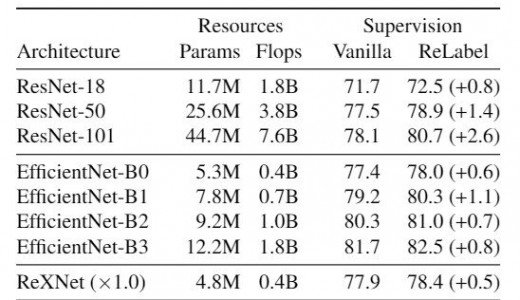Table 2: Results of applying ReLabel to multiple structures.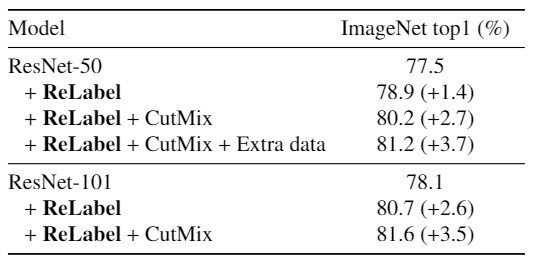Table 3: Comparison of accuracy in ImageNet.

ReLabel is a generic structure that can be used for a variety of networks using different learning methods. Here, we have experimented with ResNet-18, ResNet-101, EfficientNet-{B0, B1, B2, B3} and ReXNet. As shown in the above table, we have observed an improvement in accuracy for all the networks using ReLabel.

We also achieve SoTA in ImageNet top-1 accuracy by using ReLabel on the cropped images of CutMix algorithm in our previous work.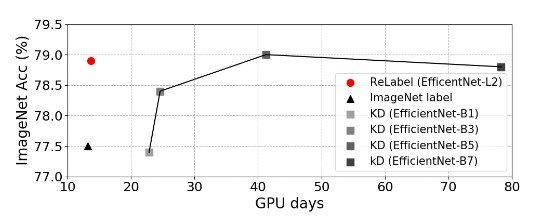Figure 7: Comparison with the distillation of knowledge

We also compared knowledge distillation (KD) with ReLabel to evaluate the learning time cost. However, we found that it takes at least three times as much time to train EfficientNet as ReLabel.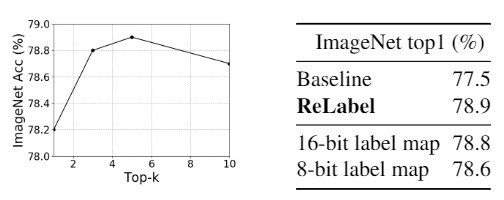Table 4: Storage vs Performance

Next, we investigate the trade-off between memory storage and performance in training with ReLabel. Normally, ReLabel experiments efficiently with top-k (k=5), but we see that as the number of data used increases, the memory is overwhelmed and the accuracy decreases. Furthermore, we found that 16-bit quantization is more accurate than 8-bit quantization when quantizing the label map.

The output image result using ReLabel is as follows.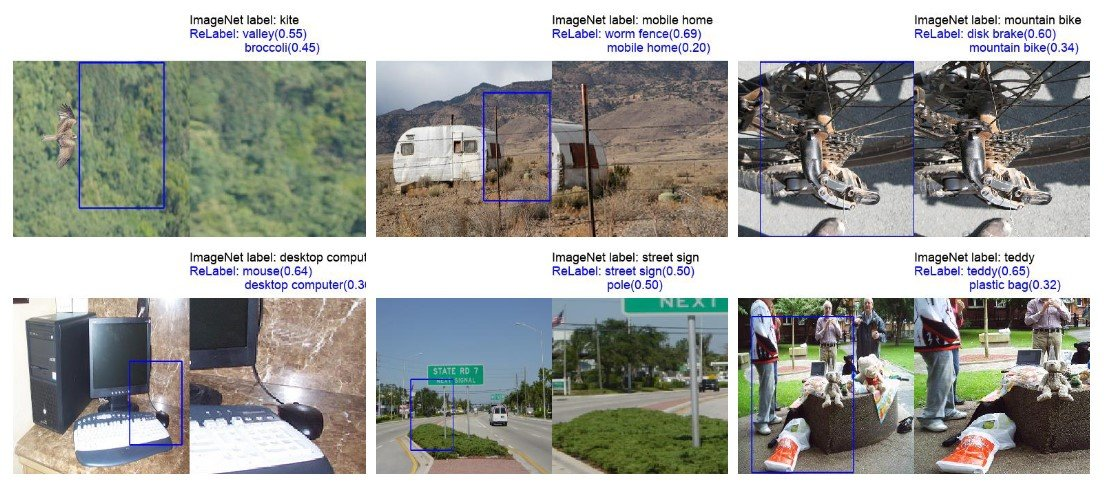Figure 8: An example of applying ReLabel using the ImageNet training set.

### transfer learning

As a standard benchmark, ImageNet is widely used as a pre-trained model. Here we experiment with transfer learning on ImageNet with ReLabel, using the COCO dataset for five fine-grained discrimination tasks, object detection, and instance segmentation tasks.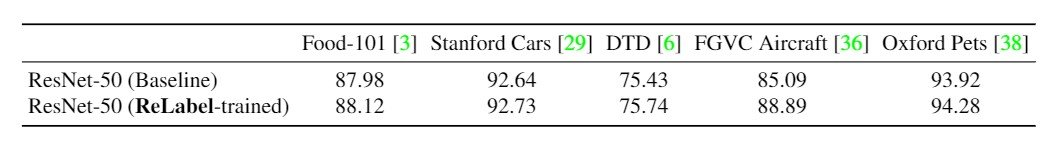Table 5: Identification results for Fine-grained.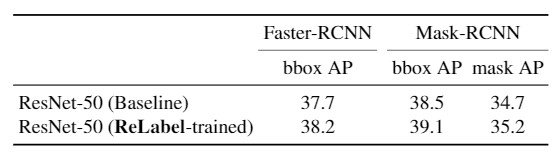Table 6: Results of object detection and instance segmentation.

We have experimented on several datasets for the discrimination task and the results are shown in the table above, and we can see that the performance of each dataset is better when trained with ReLabel. During training, we used SGD for 5000 iterations and hyperparameter tuning using grid search.

Furthermore, we can see that both Faster-RCNN and Mask-RCNN improve by 0.5 pp and average precision for the object detection and instance segmentation tasks.

### Multiple label identification

Since random cropping watering down is effective for learning multiple labels, we test whether additional local information to ReLabel and LabelPooling can make learning multiple labels more effective. To experiment, we used the COCO dataset and human-annotated data. We also investigated the effect of label map oracles using label maps created by machine learning. We then trained a multi-label discriminator using LabelPooling based on the label maps created according to the random crop coordinates.224 x 224 and 448 x 448 input images were used to feed the ResNet-50 and ResNet-101 networks, and binary cross-entropy loss was applied.

The results are shown below.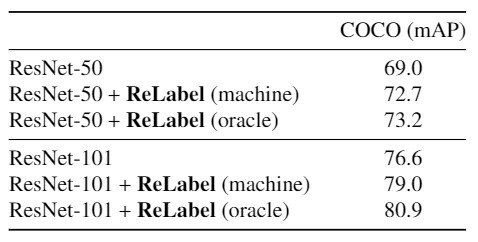Table 10: Comparison with multi-label tasks

The table shows that using ReLabel with a label map created by machine learning results in an mAP of +3.7pp for ResNet-50 and +2.4pp for ResNet-101 while using the oracle label map results in an mAP of +4.2pp for ResNet-50 and + 4.3pp on ResNet-101. This allowed us to improve the performance of ReLabel for learning location-wise multiple label identification.

## Summary

ImageNet dataset, which is prominent in image identification, labeling noise is terrible and there are multiple classes of objects while it is a single label benchmark. Therefore, in previous studies, multiple labels were annotated, but due to the significant annotation cost, the cost demand was low, and the result was that multiple objects were sometimes cropped at training time, and even random image cropping was often identified as different objects. Therefore, in this study, we proposed ReLabel for the ImageNet training set, which can convert single label training into multiple label training. We also proposed LabelPooling, which uses pixel-wise multi-label predictions before the last pooling layer in order to generate multiple labels with a more robust image recognition model and additional image data. As a result, we achieved 78.9% identification accuracy on ResNet-50 and 80.2% accuracy on ImageNet with CutMix regularization. In addition, we found that the performance is improved on transition learning and multiple benchmarks. It is expected to be applied to a variety of datasets in the future.

If you have any suggestions for improvement of the content of the article,
please contact the AI-SCHOLAR editorial team through the contact form.